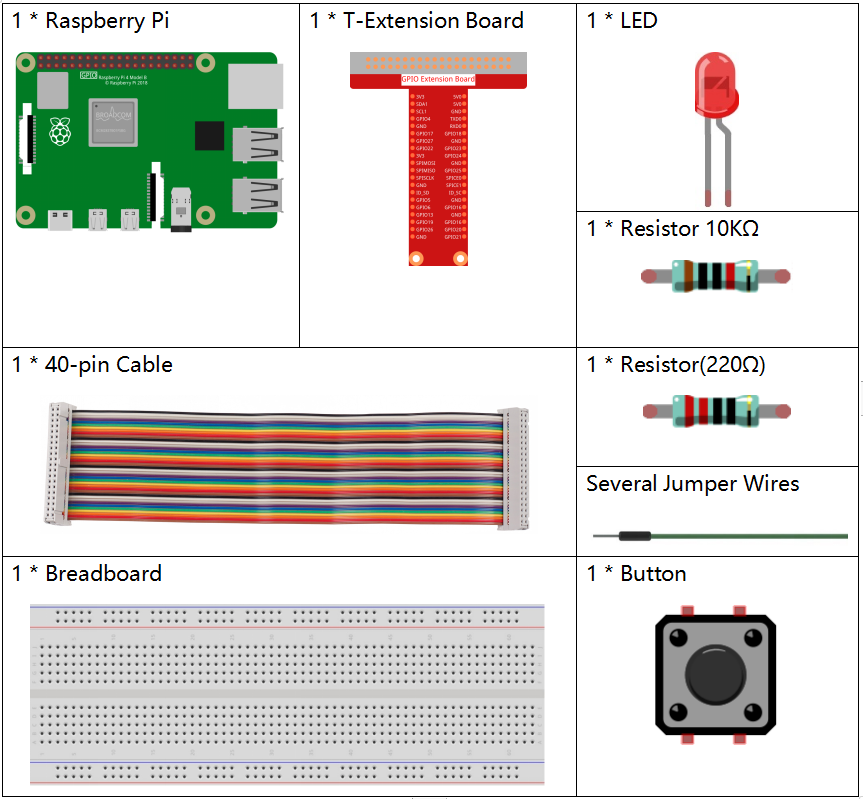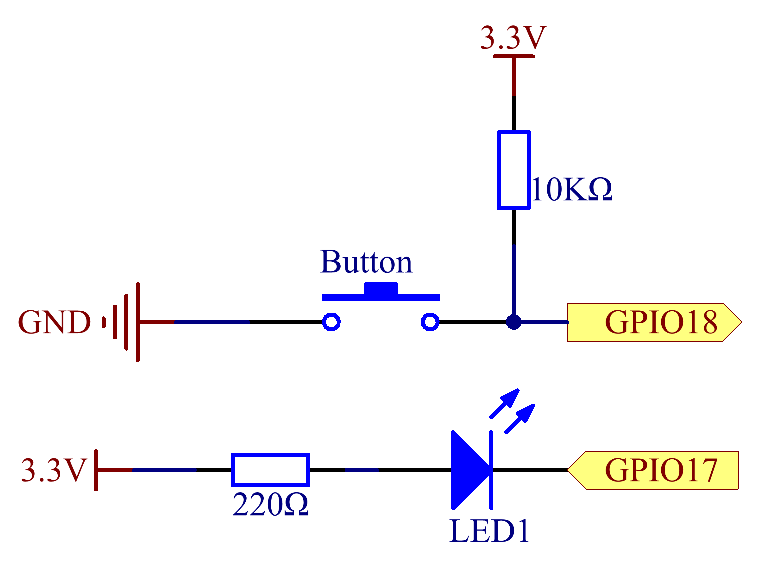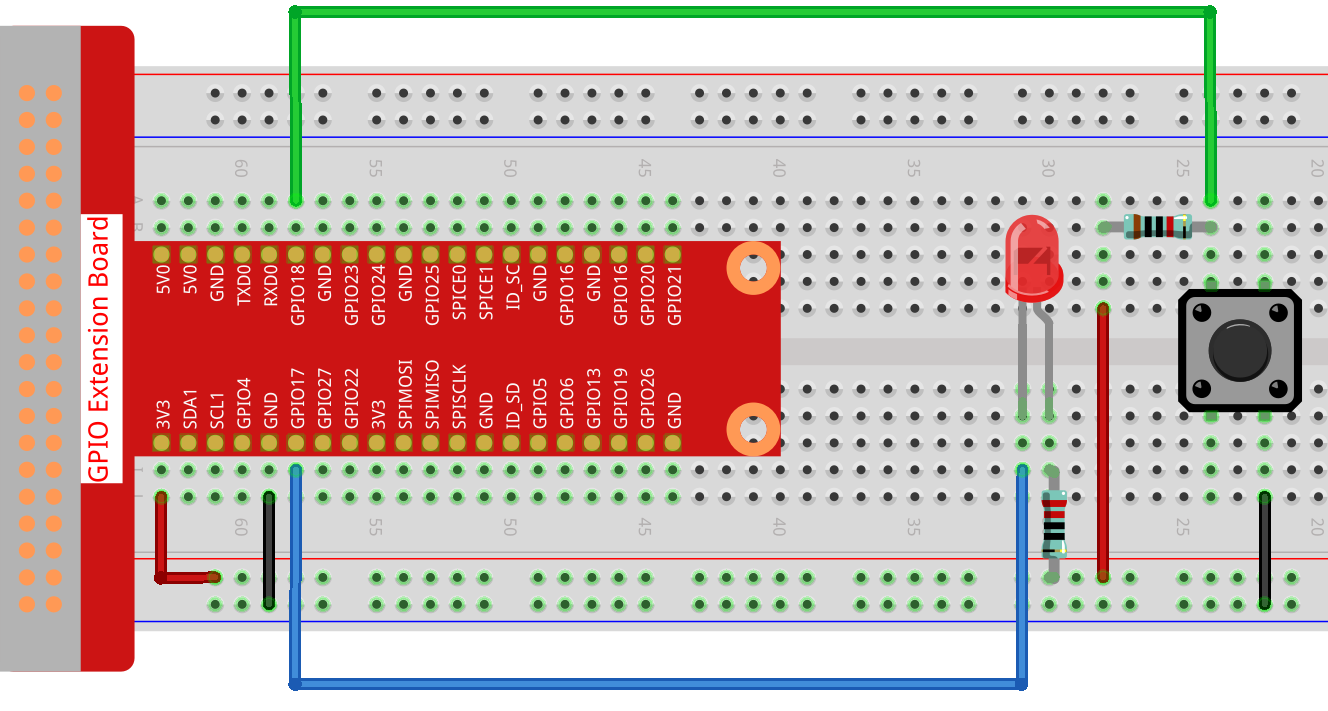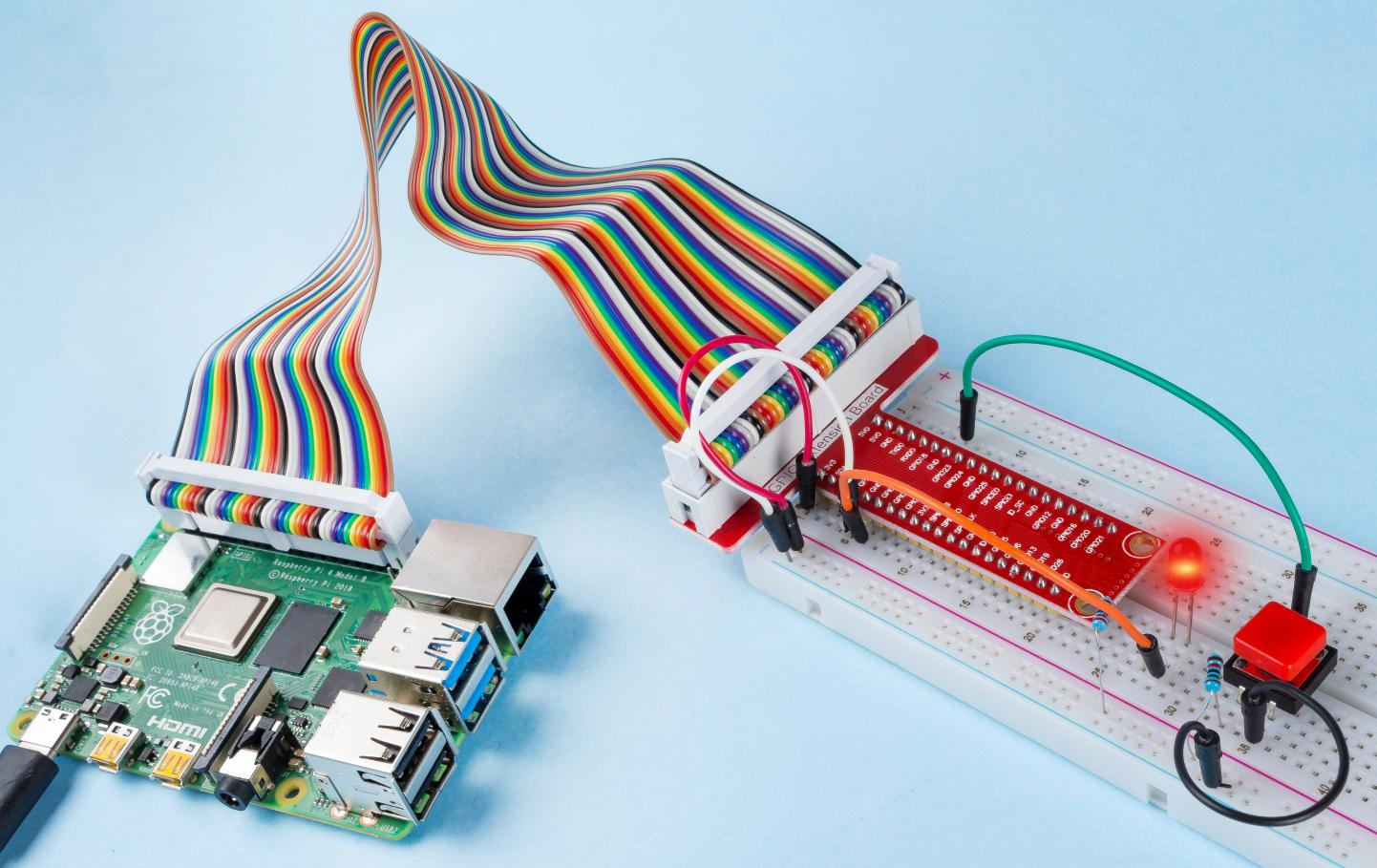# 2.1.1 Button¶

## Introduction¶

In this project, we will learn how to turn on or off the LED by using a button.

## Components¶## Schematic Diagram¶

Use a normally open button as the input of Raspberry Pi, the connection is shown in the schematic diagram below. When the button is pressed, the GPIO18 will turn into low level (0V). We can detect the state of the GPIO18 through programming. That is, if the GPIO18 turns into low level, it means the button is pressed. You can run the corresponding code when the button is pressed, and then the LED will light up.

Note

The longer pin of the LED is the anode and the shorter one is the cathode.## Experimental Procedures¶

Step 1: Build the circuit.Step 2: Go to the folder of the code.

```cd /home/pi/davinci-kit-for-raspberry-pi/nodejs/
```

Step 3: Run the code.

```sudo node button.js
```

Now, press the button, and the LED will light up; release the button, and the LED will go out.

Code

```const Gpio = require('pigpio').Gpio;

const led = new Gpio(17, {mode: Gpio.OUTPUT});

const button = new Gpio(18, {
mode: Gpio.INPUT,
pullUpDown: Gpio.PUD_DOWN,
edge: Gpio.EITHER_EDGE
});

button.on('interrupt', (level) => {
led.digitalWrite(level);
});
```

Code Explanation

```const Gpio = require('pigpio').Gpio;

const led = new Gpio(17, {mode: Gpio.OUTPUT});
```

Import the `pigpio` module, create a led object to control the IO port Gpio17, and set it to output mode.

```const button = new Gpio(18, {
mode: Gpio.INPUT,
pullUpDown: Gpio.PUD_DOWN,
edge: Gpio.EITHER_EDGE
});
```

Create a button object to control the IO port Gpio18, set it to input mode, Pull down (low when the button is not pressed, high when the button is pressed). And set the interrupt function, the mode is EITHER_EDGE, that is, both rising and falling edges will trigger the interrupt function.

```button.on('interrupt', (level) => {
led.digitalWrite(level);
});
```

Write an interrupt function, when the button is pressed, it is a falling edge, triggering the interrupt function, At this time, write the low level of the button IO port to the IO port of the led, and the led lights up.

When the button is released, it is a rising edge, triggering the interrupt function, At this time, the high level of the button IO port is written to the IO port of the led, and the led is off.

### Phenomenon Picture¶